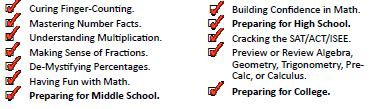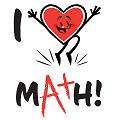* indicates a required field• phone number format invalid• email format invalidProblems detected, please review the form.
Privacy - Terms

We are open for face-to-face virtual instruction!

# News from Mathnasium of Englewood

### Summer Math Program

Feb 16, 2020June 1sr – August 31st  2020
50% off early summer registration by May 15th!

Summer is just around the corner. Do you know what your kids will be doing?  While kids may dream of days filled with swimming, ice cream, and too much screen time, parents know that a little structure goes a long way towards keeping kids motivated, happy, and safe from the academic “summer slide.”Consider including a summer math program in your plans in order to keep kids productive and learningMany families find that these programs are a great complement to other summer camps. The right summer math program can transform your child’s life with a balance of constructive learning and fun. Mathnasium’s summer program complements all camps, and flexible scheduling means it will work well with every family’s summer calendar, fitting in seamlessly around day camps or vacations. Sessions are 1 to 1.25 hours each, taken during our scheduled Monday to Thursday, and Saturday instruction times.The detailed components of each Mathnasium Power Math Workout Program are:

## Jump Starters

Jump Start:   Numerical Fluency prep for K, 1, 2nd Grades

Key curriculum areas covered in the program are:

• Addition and Subtraction Facts • Problem Solving  • Mental Math  • Patterns

• Multiplication Readiness  • Computation • Fraction Concepts

Jump Start I : Upper Elementary Math prep for 3rd, 4th Grades

This program is designed to help students transition from lower elementary (grade 3) to upper elementary (grades 4–5).

###### Key curriculum areas covered in the program are:

• Thinking in Groups    • Fraction Concepts     • Problem Solving    • Multiplication  • Algebraic Thinking    • Mental Math     • Computation   • Reading Charts and Graphs  • Patterns   • Introduction to Decimals     • Proportional Thinking  • Measurement   • Fractional Parts  •  Percent  •

Jump Start II:  Middle School Math prep for 4th, 5th, 6th Grades

This program is designed to help students transition from upper elementary (grades 4–5) to middle school (grades 6–8).

###### Key curriculum areas covered in the program are:

• Problem Solving     • Algebraic Thinking      • Mental Math     • Computation      • Fractional Parts      • Patterns  • Factors and Multiples     • LCM and GCF
• Ratio and Rate        • Proportional Thinking  • Solving Proportions        • Integer Concepts
• Adding and Subtracting Integers  • Divisibility   • Area and Perimeter  • Pre-Algebra

## Master Series

Master:  Times Tables prep for 3rd grade & up
This program is designed for students going into grade 3 and 4 and up who want to master their Times Tables.

Key curriculum areas covered in the program are
• Counting in Equal Groups      • Multiplication as Repeated Addition      • Visual Arrays
• Word Problems involving Multiplication Facts    • Using Doubles Facts     • Using Launching Points

Master:  Fraction Concepts and Skills prep for 4th grade & up
This program is designed for students going into 4th grade and up who need a comprehensive look at Fraction Concepts.
Key curriculum areas covered in the program are
• Naming Fractions • Drawing Fractions      • Proper Fractions          • Improper Fractions    • Unity     • Mixed Numbers    • SAMEness     • Complements to the Whole   • Comparing to a Whole
• Comparing Fractions     • Ordering Fractions     • Combining Halves and Quarters
• Adding and Subtracting Fractions      • Problem Solving with Fractions

Master:   Decimal Concepts and Skills prep for 4th grade & up
This program is designed for students going into 5th grade and up who need a comprehensive look at Decimal Concepts and Skills.
Key curriculum areas covered in the program are
• Place Value of Whole Numbers     • The Meaning of the Decimal Point    • Place Value of Decimals • Drawing Decimals     • Connection Between Common Fractions and Decimal Fractions                   • Finding Numbers Between Decimals     • Ordering Decimals     • Counting by Decimals                 • Rounding off Decimals     • Thinking in 10s    • Multiplying and Dividing Decimals by Powers of 10
• Adding, Subtracting, Multiplying, and Dividing Decimals    • Problem Solving With Decimals

Master:   Percent (basic) prep 5rd grade & up
This program is designed for students going into 5th grade and up who want a comprehensive introduction to the concepts and skills involving Percents.
Key curriculum areas covered in the program are
• Special Percents     • Percent—Type 1: Finding the Part      • Building 100%      • Percent—Type 2: Finding the Whole    • Changing Fractions to Decimals to Percents     • Percent—Type 3: Finding the Percent(age)     • Getting Ready for More Advanced Work with Percent

This program is designed for students going into 7th grade and up who want to do advanced work with Percents.
Key curriculum areas covered in the program are
• Special Percents     • Doing Percent Mentally      • Building 100%      • Changing Fractions to Decimals to Percents      • Percent—Type 1: Finding the Part (with equations)      • Percent—Type 2: Finding the Whole (with equations)      • Percent—Type 3: Finding the Percent(age) (with equations)     • Percent—Type 1: Finding the Part (word problems)      • Percent—Type 2: Finding the Whole (word problems)     • Percent—Type 3: Finding the Percent(age) (word problems)               • Percent—Type 4: Over 100% (word problems) • Percent—Type 5: Under 100% (word problems)     • Percent—Extended Practice • The Ultimate Percentage Questions

## Power Intros

A Power Math Intro:  Pre-Algebra prep for 6th grade & up
This program is designed for students who will soon be starting Pre– Algebra and need a review of the critical concepts and skills necessary for success in the course.

Key curriculum areas covered in the program are
• Mixed Numbers     • SAMEness      • Fractional Parts      • Wholes and Parts   • Proportional Thinking     • Percent     • Measurement     • Problem Solving
• Computation with Fractions     • Comparing and Ordering Fractions     • Denominate Numbers

A Power Math Intro:  Algebra I Readiness prep for 6th , 7th grades & up
This program is designed for students who will soon be starting Algebra 1 and need a review of the critical concepts and skills necessary for success in the course.

Key curriculum areas covered in the program are:
• Order of Operations     • Absolute Value     • Adding and Subtracting Integers       • Evaluating Algebraic Expressions    • Distributive Property     • Algebra Vocabulary    • SAMEness (combining similar terms)    • Multiplying and Dividing Monomials    • Integer Exponents    • Algebraic Thinking (problem solving)    • Function Charts    • Solving Linear Equations    • Writing and Solving Mathematical Equations • Review of Percents and Fractional Parts

A Power Math Intro:  High School Geometry Readiness prep for 8th grade & up
This program is designed for students who will be starting High School Geometry and need a review of the critical concepts and skills necessary for success in the course.

Key curriculum areas covered in the program are
• Vocabulary of Geometry   • Polygons    • Lines, Rays, and Segments    • Angles    • Points, Lines, Planes, and Space    • Congruent and Similar Figures    • Triangles    • Circles   • Area, Perimeter, and Volume    • The Pythagorean Theorem    • Introduction to Synthetic Geometry   • The Concept of a Geometric Proof   • Formulas

## Power Reviews

A Power Math Review (Algebra II Prep):   Algebra I: 8th grade & up
This program is designed for students who have completed first year Algebra and need a review of the entire course. It is not a preview of Algebra II, but would serve as a good review for most students who are planning on taking Algebra II in the fall.
Key curriculum areas covered in the program are
• Types of Number Systems • The Meaning of Slope • Direct, Indirect, and Joint Variation • Standard Form and Slope-Intercept Form • Writing Linear Equations • Graphing Linear Equations • Factoring by “Pulling Out” • Factoring “In Groups” • Factoring Quadratic Equations [basic] • Factoring Quadratic Equations [advanced] • Factoring the Difference of Squares • Squaring Binomials • Recognizing Perfect Square Trinomials • Solving Quadratic Equations by Factoring • Simplifying Radicals • Factoring by Completing the Square • Rational Expressions (the “–1 Trick”) • Operations with Rational Expressions (x) • Systems of Linear Equations • All About Functions • The Laws of Functions • Combining Radicals • Solving Quadratic Equations by Using the Quadratic Formula • The Nature of the Roots of Quadratic and Cubic Equations • Operations Expressed with Rational Expressions (+ & –) • Writing Mathematical Models and Expressions

A Power Math Review:  High School Geometry: 8th grade & up
This program is designed for students who have taken High School Geometry and need a review of the critical concepts and skills from the course.
Key curriculum areas covered in the program are
All Areas of High School Geometry